Next: The GradientNonLinear procedure Up: Solving systems with linear Previous: Example   Contents

This procedure may be used if the gradient of the equations are available. A full version is implemented as:

INTEGER_VECTOR TypeEq,
INTERVAL_VECTOR (* IntervalFunction)(int,int,INTERVAL_VECTOR &),
void (* NonLinear)(INTERVAL_VECTOR &x,INTERVAL_VECTOR &X),
void (* CoeffLinear)(MATRIX &U),
double MaxDiam,
int FullSimplex,
INTERVAL_VECTOR & TheDomain,
int Order,int Iteration,int Stop,
double epsilon,double epsilonf,double Dist,
INTERVAL_MATRIX & Solution,
INTEGER_MATRIX &GI,
int (* Simp_Proc)(INTERVAL_VECTOR &))
the arguments being:
• m: number of unknowns
• n: number of equations, see the note 2.3.4.1
• NbNl: number of equations that have no linear term at all or are inequalities.
• TypeEq: an array of integers that indicate the type for the equations. TypeEq(i) is -1,0,1 if equation i is an inequality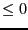, an equation or an inequality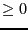.
• IntervalFunction: a function which return the interval vector evaluation of the equations, see the note 2.3.4.3 on how to write this procedure. The equations must be ordered: first the equations with linear terms then the equations without any linear terms and finally the inequalities
• Gradient: a procedure which return the Jacobian matrix of the system for given values of the unknowns (see note 2.4.2.2)
• NonLinear: a procedure to compute the non linear part of the equations, see note 2.14.2.1
• GradientNonLinear: a procedure that returns the gradient of the non linear part of the equations, see note 2.14.3.1
• CoeffLinear: a procedure that return a matrix which contain the constant coefficients of the linear term in the equation, see note 2.14.2.2
• MaxDiam: the simplex method will not be used on boxes whose maximal width is lower than this value. Should be set to 0 or a small value
• FullSimplex: this flag is used to indicate how much we will use the simplex method (which may be costly). If set to -1 only the phase I of the simplex will be used. If set to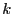with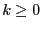, then the full simplex method will be used recursively on the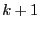variables having the largest interval width.
• TheDomain: box in which we are looking for solution of the equations
• Order: the type of order which is used to store the intervals created during the bisection process. This order may be either MAX_FUNCTION_ORDER or MAX_MIDDLE_FUNCTION_ORDER. See the note on the order 2.3.4.4.
• M: the maximum number of boxes which may be stored. See the note 2.3.4.5
• Stop: the possible values are 0,1,2
• 0: the algorithm will look for every solution in TheDomain
• 1: the algorithm will stop as soon as 1 solution has been found
• 2: the algorithm will stop as soon as Nb solutions have been found
• epsilon: the maximal width of the solution intervals, see the note 2.3.4.6
• epsilonf: the maximal width of the equation intervals for a solution, see the note 2.3.4.6
• Dist: minimal distance between the middle point of two interval solutions, see the note 2.3.4.7
• Solution: an interval matrix of size (Nb,m) which will contained the solution intervals. This list is sorted using the order specified by Order
• Nb: the maximal number of solution which will be returned by the algorithm
• UseGradNL: if set to 1 the algorithm will use the gradient of the non linear part of the equations to improve their interval evaluation. Otherwise must be set to 0.
• GI: an integer matrix which give a-priori information on the sign of the derivative of the function. GI(i,j) indicates the sign of the derivative of function i with respect to variable j using the following code:
• -1: the derivative is always negative
• 0: the function is not dependent of variable j
• 1: the derivative is always positive
• 2: the sign of the derivative is not known
• Simp_Proc: a user-supplied procedure that take as input the current box and proceed to some further reduction of the width of the box or even determine that there is no solution for this box, in which case it should return -1 (see note 2.3.3). Remember that you may use the 3B method to improve the efficiency of this algorithm (see section 2.3.2).
The following variables play also a role in the computation:
• ALIAS_Store_Gradient: if not 0 the gradient matrix of each box will be stored together with the boxes. Must be set to 0 for large problem (default value: 1)
• ALIAS_Diam_Max_Gradient: if the maximal width of the ranges in a box is lower than this value, then the gradient will be used to perform the interval evaluation of the functions (default value: 1.e10)
• ALIAS_Diam_Max_Kraw: if the maximal width of the ranges in a box is lower than this value, then the Krawczyk operator will be used to determine if there is a unique solution in the box (default value: 1.e10)
• ALIAS_Diam_Max_Newton: if the maximal width of the ranges in a box is lower than this value, then the interval Newton method will be used either to try to reduce the width of the box or to to ensure that there is no solution of the system in the box (default value: 1.e10)
• Min_Diam_Simplex: if the maximal width of the input box is lower than this value, then the simplex method will be used
• Max_Diam_Simplex: if the maximal width of the input box is lower than this value, then the simplex method will not be used
• Min_Improve_Simplex: when applying the simplex method if the change on one variable is larger than this value, then the simplex method will be repeated
• ALIAS_Simplex_Expanded: if set to 1 the expression has been expanded with respect to the lower bound of each variable

There are several versions of this procedure in which several arguments of the general procedure may be omitted. The following table indicates which arguments may be omitted and the corresponding assumptions (EO=equations only).

 omitted NbNl 0 0 0 TypeEq EO EO EO EO EO EO GradientNonLinear not known not known UseGradNL 0 0 0 0
In all cases you may omit the GI argument (the derivatives are assumed to be unknown) and Simp_Proc.

SubsectionsNext: The GradientNonLinear procedure Up: Solving systems with linear Previous: Example   Contents
Jean-Pierre Merlet 2012-12-20